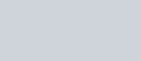To find the inverse of a 3×3 matrix A say, (Last video) you will need to be familiar with several new matrix methods first

1. Finding the minor of each element of matrix A
2. Finding the cofactor of matrix A

With these I show you how to find the inverse of a matrix A

where I use the examplePrerequisite: Finding minors of elements in a 3×3 matrix

Matrices - Minors of elements in a 3x3 matrix | ExamSolutions - maths problems answered - youtube Video

Finding the cofactor of a 3×3 matrix

Matrices - Finding the cofactor matrix | ExamSolutions - maths problems answered - youtube Video

Putting it all together – Finding the inverse of a 3×3 matrix

Matrices - Inverse of a 3x3 matrix | ExamSolutions - maths problems answered - youtube Video

Example to try

Matrices - Working with Inverse Matrices (Example) | ExamSolutions - maths problems answered - youtube Video
Inverse Matrices on a Casio ClassWiz Calculator | ExamSolutions - maths problems answered - youtube Video